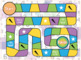# GRADE 4 Math Board Game - Review of Key CCSS points - 48 CardsSubject
Resource Type
File Type
Zip (9 MB|9 pages)
Standards
\$3.50
• Product Description
• Standards
***New Resource!***

A fun end of year activity to recap and review all of the Common Core Standards for Grade 4. Can also be used as an activity at the beginning of Grade 5 to recap the previous year.

- 6 pages of 8 cards (48 cards in total) - includes at least 8 cards of each CCSS topic (OA, NBT, NF, MD, G) covering the main standards of each. Each card has the answer at the bottom.
- A printable game board
- Instructions

A perfect way to build on both mathematical ability and team work skills!

Compare two decimals to hundredths by reasoning about their size. Recognize that comparisons are valid only when the two decimals refer to the same whole. Record the results of comparisons with the symbols >, =, or <, and justify the conclusions, e.g., by using a visual model.
Use decimal notation for fractions with denominators 10 or 100. For example, rewrite 0.62 as 62/100; describe a length as 0.62 meters; locate 0.62 on a number line diagram.
Express a fraction with denominator 10 as an equivalent fraction with denominator 100, and use this technique to add two fractions with respective denominators 10 and 100. For example, express 3/10 as 30/100, and add 3/10 + 4/100 = 34/100.
Recognize a line of symmetry for a two-dimensional figure as a line across the figure such that the figure can be folded along the line into matching parts. Identify line-symmetric figures and draw lines of symmetry.
Classify two-dimensional figures based on the presence or absence of parallel or perpendicular lines, or the presence or absence of angles of a specified size. Recognize right triangles as a category, and identify right triangles.
Total Pages
9 pages